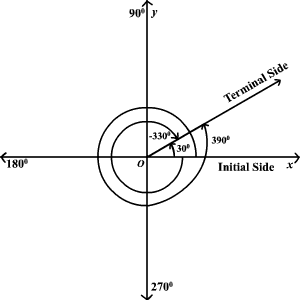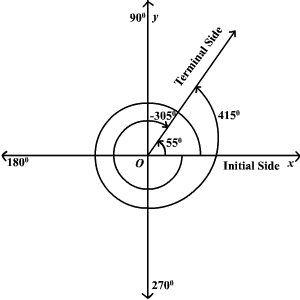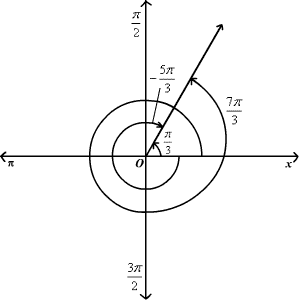# Coterminal Angles

Coterminal angles are angles in standard position (angles with the initial side on the positive-axis) that have a common terminal side.  For example,andare all coterminal.To find a positive and a negative angle coterminal with a given angle, you can add and subtractif the angle is measured in degrees orif the angle is measured in radians .

Example 1:

Find a positive and a negative angle coterminal with aangle.Aangle and aangle are coterminal with aangle.Example 2:

Find a positive and a negative angle coterminal with aangle.Aangle and aangle are coterminal with aangle.